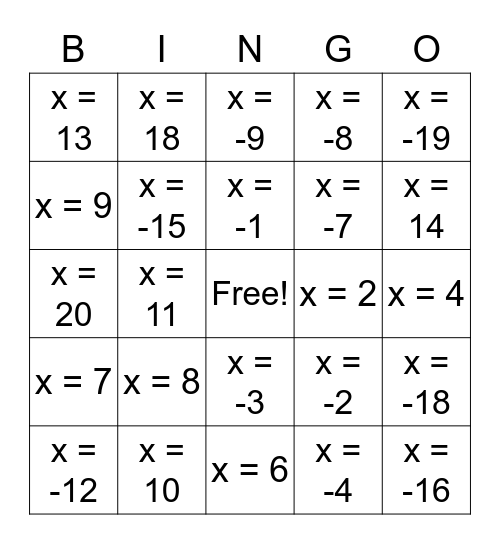# Solving Equations BingoThis bingo card has a free space and 28 words: x = -18, x = -6, x = -7, x = -4, x = 9, x = -9, x = 18, x = 7, x = 2, x = -12, x = 20, x = -8, x = 10, x = 6, x = 14, x = -13, x = 8, x = -15, x = -1, x = -3, x = 13, x = 1, x = -16, x = 11, x = 4, x = -2, x = -19 and x = 12.

## Play Online